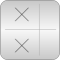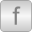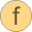# ComplexMath

Library of complex mathematical functions (e.g., sin, cos) and of functions operating on complex vectors and matrices# Package ContentsVectors Library of functions operating on complex vectorssin Sine of complex numbercos Cosine of complex numbertan Tangent of complex numberasin Arc-sine of complex numberacos Arc-cosine of complex numberatan Arc-tangent of complex numbersinh Hyperbolic-sine of complex numbercosh Hyperbolic-cosine of complex numbertanh Hyperbolic-tangent of complex numberasinh Area-hyperbolic-sine of complex numberacosh Area-hyperbolic-cosine of complex numberatanh Area-hyperbolic-tangent of complex numberexp Exponential of complex numberlog Logarithm of complex number'abs' Absolute value of complex numberarg Phase angle of complex numberconj Conjugate of complex numberreal Real part of complex numberimag Imaginary part of complex numberfromPolar Complex from polar representation'sqrt' Square root of complex number'max' Return maximum element of complex vector'min' Return minimum element of complex vector'sum' Return sum of complex vector'product' Return product of complex vector

# Package Constants (1)

j Value: Complex(0, 1) Type: Complex Description: Imaginary unit

# Information

This information is part of the Modelica Standard Library maintained by the Modelica Association.

This package contains basic mathematical functions operating on complex numbers (such as sin(..)), as well as functions operating on vectors of complex numbers.